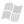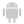WINDEV, WEBDEV AND WINDEV MOBILEONLINE HELPVersion: 15161718192021222324
 Home |Sign in | EnglishHelpWLanguageWLanguage functionsControls, pages and windowsPivot Table functionsObject syntaxPVTCalculateUpdatePresentationExampleCalculation modeWhen to use PVTCalculateUpdate?See alsoConstants for managing the pivot tablesFunctions for managing the Pivot Table controlsPVTAxisXYPVTCalculateAllPVTCalculateUpdatePVTCollapsePVTCollapseAllPVTExpandPVTExpandAllPVTFilterPVTHeaderValuePVTInfoXYPVTListPositionHeaderPVTLoadPVTSavePVTSelectPVTSelectOccurrencePVTToExcelpvtPosition
 Calculation modeWhen to use PVTCalculateUpdate? WINDEVWEBDEVWINDEV MobileOthersPVTCalculateUpdate (Function) In french: TCDCalculeMiseAJour Updates some of the data found in a Pivot Table control from a given date. Notes: The content of the Pivot Table control was previously loaded into memory using PVTLoad. The Pivot Table control includes a Date column, row or display filter. Example // Builds the file namesFile = SysDir(srAppData) + ["\"] + "StatsByCountry.tcd" // The file exists?IF fFileExist(sFile) THEN // Yes, load it PVTLoad(PVT_Statistics, sFile) // Update the Pivot Table control from the last calculation date // (we assume that the records associated with an earlier date // have not been modified) PVTCalculateUpdate(PVT_Statistics, LoadParameter(DATE_LAST_CALCULATION))ELSE // The file does not exist, calculate it PVTCalculateAll(PVT_Statistics)END Syntax PVTCalculateUpdate( [, ]) : Control nameName of Pivot Table control to use. : Optional character string or Date variableDate from which the content of the Pivot Table control must be recalculated. This date must be in "YYYYMMDD" format. All the records associated with a Date item whose content corresponds to a date greater than will be taken into account.If this parameter is not specified, the date taken into account corresponds to the maximum date of the last result calculated or read by PVTLoad. Remarks Calculation modeThe records taken into account for the re-calculation have the following characteristics: The records include a Date item. The content of the Date item is greater than or equal to the value of . The former values found in the Pivot Table control (if any) are deleted from the Pivot Table control from .The calculation can be quite long if an important volume of data must be read. In this case, a progress bar is displayed in the control.At the end of calculation, the new Pivot Table is displayed. Then, the result can be saved by PVTSave. When to use PVTCalculateUpdate?PVTCalculateUpdate must be used when the data is fixed: invoices for example. You only have the ability to add new invoices. In this case, the recalculation date corresponds to the date on which the content of the Pivot Table control was saved. If the data displayed in the Pivot Table control is modifiable (a quantity, a price, etc.), you must:: entirely recalculate the Pivot Table control. recalculate the Pivot Table control from the date corresponding to the modified record. Component : wd240obj.dll Minimum version required Version 18 This page is also available for…